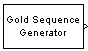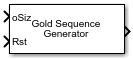# Gold Sequence Generator

Generate Gold sequence from set of sequences

•Libraries:
Communications Toolbox / Comm Sources / Sequence Generators

## Description

The Gold Sequence Generator block generates a binary sequence with small periodic cross-correlation properties from a bounded set of sequences. For more information, see Gold Sequences.

These icons shows the block with all ports enabled.## Ports

### Input

expand all

Current output size, specified as a scalar or a two-element row vector. The second element of the vector must be `1`.

Example: `[10 1]` indicates the current output column vector will be of size 10-by-1.

#### Dependencies

To enable this port select the Output variable-size signals parameter and set Maximum output size source to ```Dialog parameter```.

Data Types: `double`

Reference input signal, specified as a scalar, column vector.

#### Dependencies

To enable this port select the Output variable-size signals parameter and set Maximum output size source to ```Inherit from reference input```.

Data Types: `double`

Reset signal, specified in one of these forms.

• When the output size is variable specify as a scalar.

• Otherwise, specify as a scalar or a 2-D column vector with a length equal to Samples per frame.

The output signal resets for nonzero Rst input values. For more information, see Reset Behavior

#### Dependencies

To enable this port, select the Reset on nonzero input parameter.

Data Types: `double`

### Output

expand all

Output signal, returned as a binary column vector. At least one element of the Initial states (1) or Initial states (2) vector must be nonzero in order for the block to generate a nonzero sequence.

Data Types: `double`

## Parameters

expand all

First sequence polynomial, specified in one of these forms.

• Character vector or string scalar of a polynomial whose constant term is `1`. For more information, see Representation of Polynomials in Communications Toolbox.

• Binary-valued row vector that represents the coefficients of the polynomial in order of descending powers. The length of this vector must be N + 1, where N is the degree of the polynomial. The first and last entries must be `1`, indicating the leading term with degree N and a constant term of 1.

• Integer-valued row vector of elements that represent the exponents for the nonzero terms of the polynomial in order of descending powers. The last entry must be `0`, indicating a constant term of 1.

This property determines the feedback connections for the shift register of the first preferred PN sequence generator. The degree of the first generator polynomial must equal the degree of the second generator polynomial specified by the Preferred polynomial (2) parameter. For more information, see Preferred Pairs of Sequences.

Example: `'z^8 + z^2 + 1'`, ```[1 0 0 0 0 0 1 0 1]```, and `[8 2 0]` represent the same polynomial, p(z) = z8 + z2 + 1.

Initial states of the shift register for first sequence polynomial of the preferred pair, specified as a binary vector with length equal to the degree of Preferred polynomial (1).

Note

For the block to generate a nonzero sequence, at least one element of the initial conditions for the first or second preferred PN sequence generator must be nonzero. Specifically, the initial state of at least one of the shift registers must be nonzero.

Second sequence polynomial, specified in one of these forms.

• Character vector or string scalar of a polynomial whose constant term is `1`. For more information, see Representation of Polynomials in Communications Toolbox.

• Binary-valued row vector that represents the coefficients of the polynomial in order of descending powers. The length of this vector must be N + 1, where N is the degree of the polynomial. The first and last entries must be `1`, indicating the leading term with degree N and a constant term of 1.

• Integer-valued row vector of elements that represent the exponents for the nonzero terms of the polynomial in order of descending powers. The last entry must be `0`, indicating a constant term of 1.

This property determines the feedback connections for the shift register of the first preferred PN sequence generator. The degree of the second generator polynomial must equal the degree of the first generator polynomial specified by the Preferred polynomial (1) parameter. For more information, see Preferred Pairs of Sequences.

Example: `'z^8 + z^2 + 1'`, ```[1 0 0 0 0 0 1 0 1]```, and `[8 2 0]` represent the same polynomial, p(z) = z8 + z2 + 1.

Initial states of the shift register for second sequence polynomial of the preferred pair, specified as a binary vector with length equal to the degree of Preferred polynomial (2).

Note

For the block to generate a nonzero sequence, at least one element of the initial conditions for the first or second preferred PN sequence generator must be nonzero. Specifically, the initial state of at least one of the shift registers must be nonzero.

Sequence index of the output sequence from the set of sequences, specified as an integer scalar in the range [–2, 2n–2]. n is the degree of the preferred polynomials. For more information, see Gold Sequences.

Offset of Gold sequence from the initial time, specified as an integer scalar.

Select this parameter to enable variable-length output sequences during simulation. When you clear this parameter, the block outputs fixed-length sequences. When you select this parameter, the block can output variable-length sequences. For information about variable-size signals, see Variable-Size Signal Basics (Simulink).

Select how to specify the maximum sequence output size.

• `Dialog parameter` — Select this value to configure the block to use the Maximum output size parameter setting as the maximum permitted output sequence length. The oSiz input port specifies the current size of the output signal, and the block output inherits the sample time from the input signal. The input value of oSiz must be less than or equal to the Maximum output size parameter.

• `Inherit from reference port` — Select this value to enable the Ref input port and configure the block to inherit the sample time, maximum size, and current output size from the variable-sized signal at the Ref input port. These set the maximum permitted output sequence length.

#### Dependencies

To enable this parameter, select Output variable-size signals.

Specify the maximum output size for the block. n is a positive scalar.

Example: `[10 1]` specifies a 10-by-1 maximum size for the output signal.

#### Dependencies

To enable this parameter, select Output variable-size signals and set Maximum output size source to `Dialog parameter`.

Positive scalars specify the time in seconds between each sample of the output signal. If you set the sample time to `-1`, the output signal inherits the sample time from downstream. For information on the relationship between the Sample time and Samples per frame parameters, see Sample Timing.

#### Dependencies

To enable this parameter do not select Output variable-size signals.

Samples per frame, specified as a positive integer indicating the number of samples per frame in one channel of the output data. For information on the relationship between Sample time and Samples per frame, see Sample Timing.

#### Dependencies

To enable this parameter do not select Output variable-size signals.

Select this parameter to enable the Rst port. When a nonzero value is input at the Rst port, the internal shift registers are reset to the original values of the Initial states (1) and Initial states (2) parameters.

Output data type, specified as `boolean`, `double`, or ```Smallest unsigned integer```.

When set to `Smallest unsigned integer`, the output data type is selected based on the settings used in the Hardware Implementation Pane (Simulink) of the Configuration Parameters dialog box of the model. If ASIC/FPGA is selected in the Hardware Implementation pane, the output data type is the ideal minimum one-bit size (ufix(1)). For all other selections, it is an unsigned integer with the smallest available word length large enough to fit one bit, usually corresponding to the size of a char (uint8).

## Block Characteristics

 Data Types `Boolean` | `double` | `fixed point` Multidimensional Signals `no` Variable-Size Signals `yes`